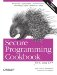# Computing the Fast Fourier Transform

Problem

You want to compute the Discrete Fourier Transform (DFT) efficiently using the Fast Fourier Transform (FFT) algorithm.

Solution

The code in Example 11-33 provides a basic implementation of the FFT.

Example 11-33. FFT implementation

```#include
#include
#include
#include

using namespace std;

unsigned int bitReverse(unsigned int x, int log2n) {
int n = 0;
for (int i=0; i < log2n; i++) {
n <<= 1;
n |= (x & 1);
x >>= 1;
}
return n;
}

const double PI = 3.1415926536;

template
void fft(Iter_T a, Iter_T b, int log2n)
{
typedef typename iterator_traits::value_type complex;
const complex J(0, 1);
int n = 1 << log2n;
for (unsigned int i=0; i < n; ++i) {
b[bitReverse(i, log2n)] = a[i];
}
for (int s = 1; s <= log2n; ++s) {
int m = 1 << s;
int m2 = m >> 1;
complex w(1, 0);
complex wm = exp(-J * (PI / m2));
for (int j=0; j < m2; ++j) {
for (int k=j; k < n; k += m) {
complex t = w * b[k + m2];
complex u = b[k];
b[k] = u + t;
b[k + m2] = u - t;
}
w *= wm;
}
}
}

int main( ) {
typedef complex cx;
cx a[] = { cx(0,0), cx(1,1), cx(3,3), cx(4,4),
cx(4, 4), cx(3, 3), cx(1,1), cx(0,0) };
cx b;
fft(a, b, 3);
for (int i=0; i<8; ++i)
cout << b[i] << "
";
}```

The program in Example 11-33 produces the following output:

```(16,16)
(-4.82843,-11.6569)
(0,0)
(-0.343146,0.828427)
(0,0)
(0.828427,-0.343146)
(0,0)
(-11.6569,-4.82843)```

Discussion

The Fourier transform is an important equation for spectral analysis, and is required frequently in engineering and scientific applications. The FFT is an algorithm for computing a DFT that operates in N log2(N) complexity versus the expected N2 complexity of a naive implementation of a DFT. The FFT achieves such an impressive speed-up by removing redundant computations.

Finding a good FFT implementation written in idiomatic C++ (i.e., C++ that isn't mechanically ported from old Fortran or C algorithms) and that isn't severely restricted by a license is very hard. The code in Example 11-33 is based on public domain code that can be found on the digital signal processing newswgoup on usenet (comp.dsp). A big advantage of an idiomatic C++ solution over the more common C-style FFT implementations is that the standard library provides the complex template that significantly reduces the amount of code needed. The fft( ) function in Example 11-33, was written to be as simple as possible rather than focusing on efficiency.Secure Programming Cookbook for C and C++: Recipes for Cryptography, Authentication, Input Validation & More
ISBN: 0596003943
EAN: 2147483647
Year: 2006
Pages: 241